An article in the July 30, 2016 New York Times argues that high stock prices are the result of low bond yields. To paraphrase the article: The S&P 500 index has a dividend yield of 2.1%, 40% higher than the 1.5% yield on 10-year Treasury notes. Investors have moved to stocks, chasing yield.
This post explains why dividend yield and bond yield are not substitutes.

# Index Dividends and 10-Year Treasury yields

I agree with the first half of the argument - Over the past 15 years, the yield on 10-year Treasury notes has declined, while the dividend yield on the S&P 500 index has (on average) increased: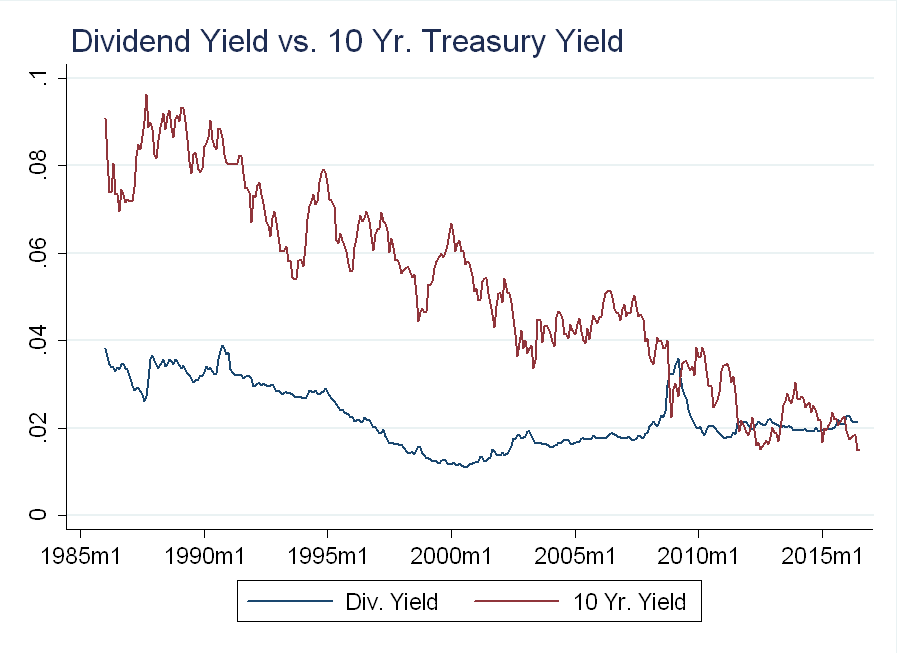This doesn’t mean you should sell your bonds and buy stocks. More important is the total return - the sum of yield and capital gains (i.e. the price going up).

# Dividend Yield

Dividend yield, $D/P$, can increase for two reasons - either dividends go up, or prices go down:At the index level, dividends don’t move that much and changes in dividends are weakly correlated with dividend yield.
There is, however, a strong inverse relationship between dividend yield and index price level:The standard deviation of dividend growth is about one eighth the standard deviation of stock returns, so the magnitude of price changes drowns out changes in dividends: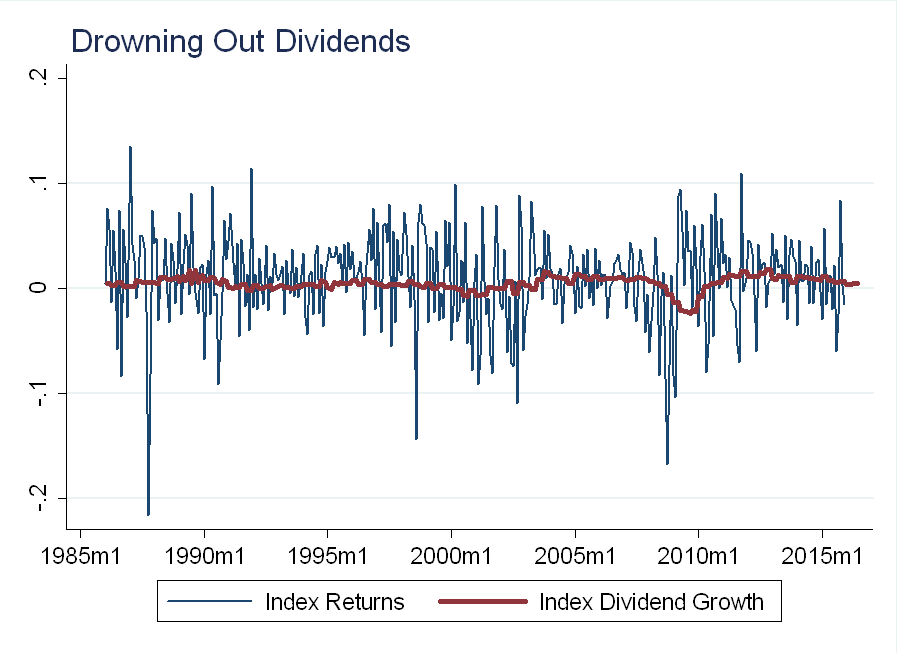The point is - dividends have been stable, but the price of the underlying index has not.

# 10-Year Treasury Yield

Yields on existing bonds can increase for one reason - the price went down. This is because (assuming the U.S. government always pays its debts), you are guaranteed to get the face value for a Treasury note at maturity. There is no such guarantee with stocks.
This doesn’t mean bonds are easier to understand than stocks, as prices can change for many reasons. For example: if interest rates rise, existing bonds go down in price (suppose not, then arbitrageurs would short old bonds, and buy new bonds to earn the difference in yields).
Compare the price of 10-year bonds to S&P 500 index. Both series are normalized to 100 in January, 1986:This doesn’t mean you would have earned zero buying the 10-year notes. Below, I plot the total return (assuming you reinvest dividends and reinvest coupon payments) for each asset: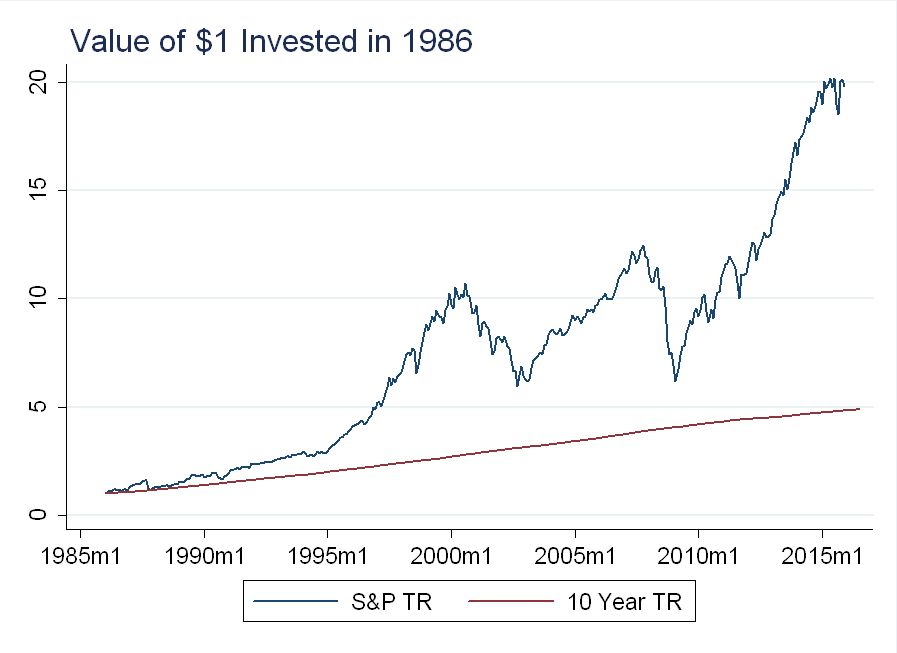The bond investment goes up a little every year, while the stock investment loses nearly 50% of its value twice in the last 30 years. Despite this, the stocks would be worth 4x as much today as the bonds!

# Timing

In a two period asset pricing model: \begin{equation} P_t= E_t \left(\beta \frac{u’(c_{t+1})}{u’(c_t)} x_{t+1} \right) \end{equation} With risk averse investors, an asset whose payoff, $x_{t+1}$, covariates negatively with consumption commands a higher price (and a lower return) than an asset that covaries positively with consumption. This is why people buy insurance: It’s good to get a check when your house burns down. In the financial crisis, bond prices went up (yields went down), while stock prices went down:This is another reason to treat dividend yields and bond yields differently - if you want to hedge against losing your job in a recession, bonds are a better bet (whether job loss is at the extensive or intensive margin during recessions will be the topic of a future blog post).

# Individual Stocks

Let’s look at two dividend paying stocks mentioned in the article, Verizon and AT&T (formerly SBC). We see the same pattern as we did for the index - dividends are relatively stable, while prices are not: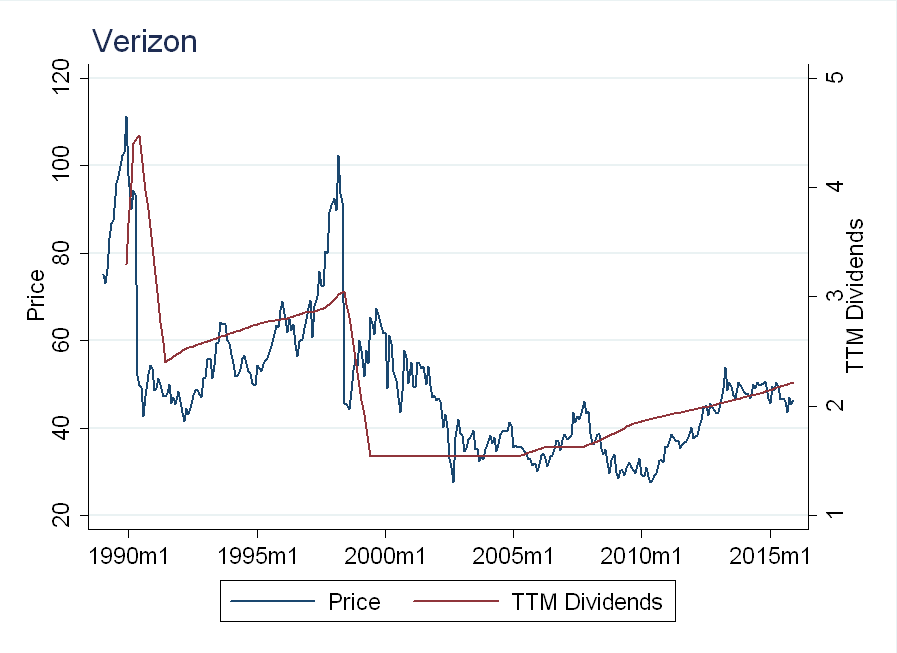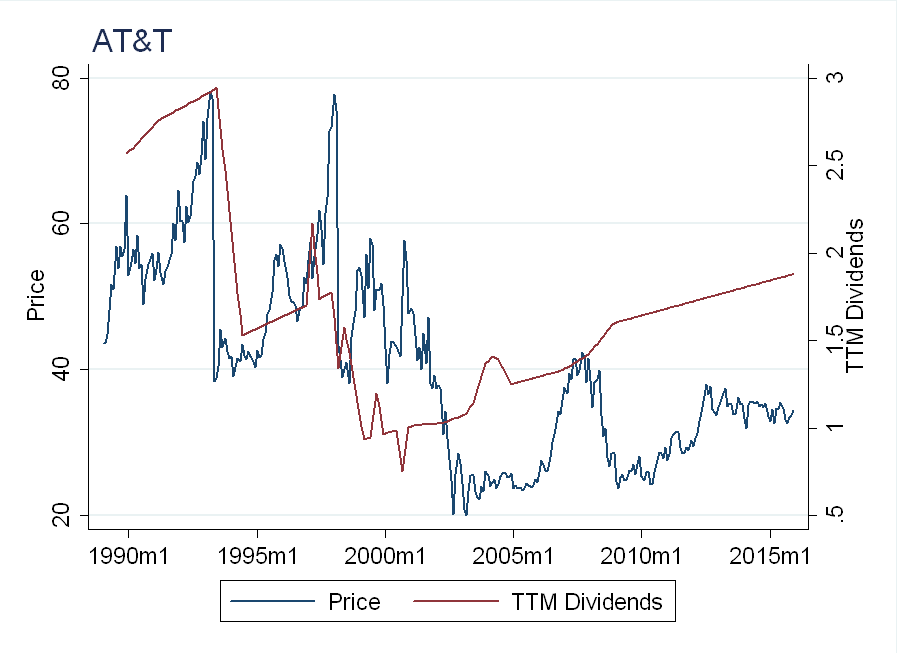Investors might be attracted to these stocks because their dividend yields are now more than double the yield on 10-Year Treasury Notes: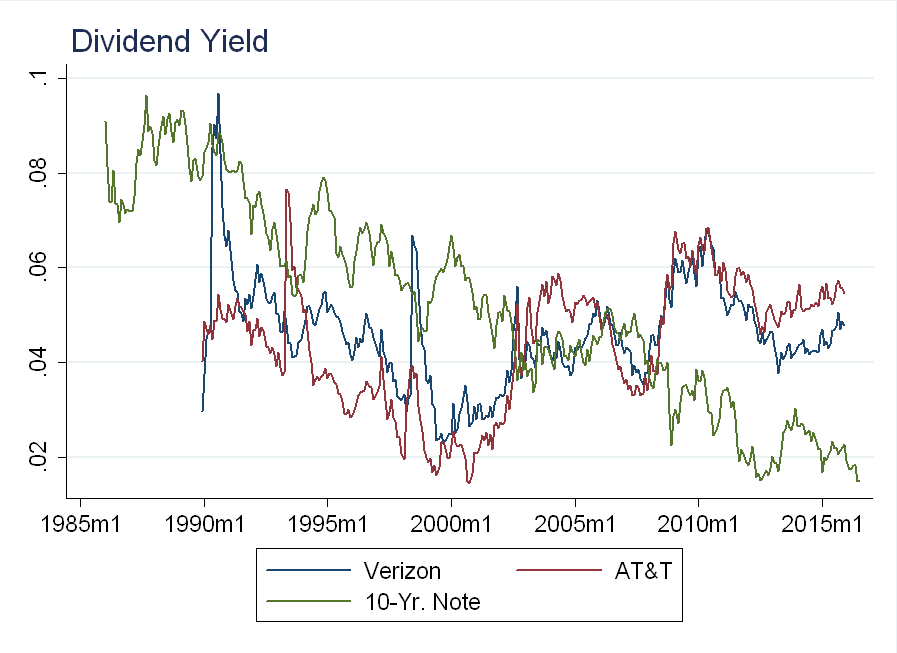It’s important to remember, however, that both these stocks lost over 30% of their value during the financial crisis. If you were forced to sell, (for example, owing to liquidity constraints) you would have taken a big loss, lowering the effective yield below that of the 10-year note.

# Conclusion

There’s something missing from this analysis - the relationship between inflation, bond yields and stock prices. Mild inflation will affect real bond returns more than real stock returns. This omission, however, doesn’t change the main result - even though dividend yields are relatively stable over time, there is no guarantee on the value of the underlying asset. Investors may be able to increase yields 40% by switching from bonds to stocks, but not without substantial additional risk.